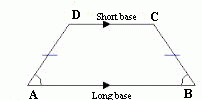### Isosceles Trapezoid

The Isosceles Trapezoids is a quadrilateral with two non parallel sides equal and two parallel sides unequal. four interior angles, totaling 360 degrees.
The non parallel sides are called sides or legs, while the two parallel sides are called bases, one short and the other long.
Like, any trapezium, the Isosceles trapezoid has as follow:
a) Area;
b) Perimeter: sum of the lengths of the bases and sides;
c) Height: perpendicular line segment from a base to the other;
d) Midsegment: line segment joining the midpoints of two sides;
e) Diagonal: straight line segment that connect the opposite vertices.
To calculate the elements of the iso trapezoid; enter the two bases, a side or height. Use the point as decimal separator. Ex 1,300.34 enter: 1300.34. The results will be shown after a click on Calculate.Isosceles Trapezoid Decimal places: Short base: Long base: Side: Height: Perimeter: Area: Side Height: Diagonal A C: Diagonal B D: Midsegment: Angle A = B: Angle C = D: Note:

Note: The accuracy of the calculator and its applicability to particular cases is not guaranteed.